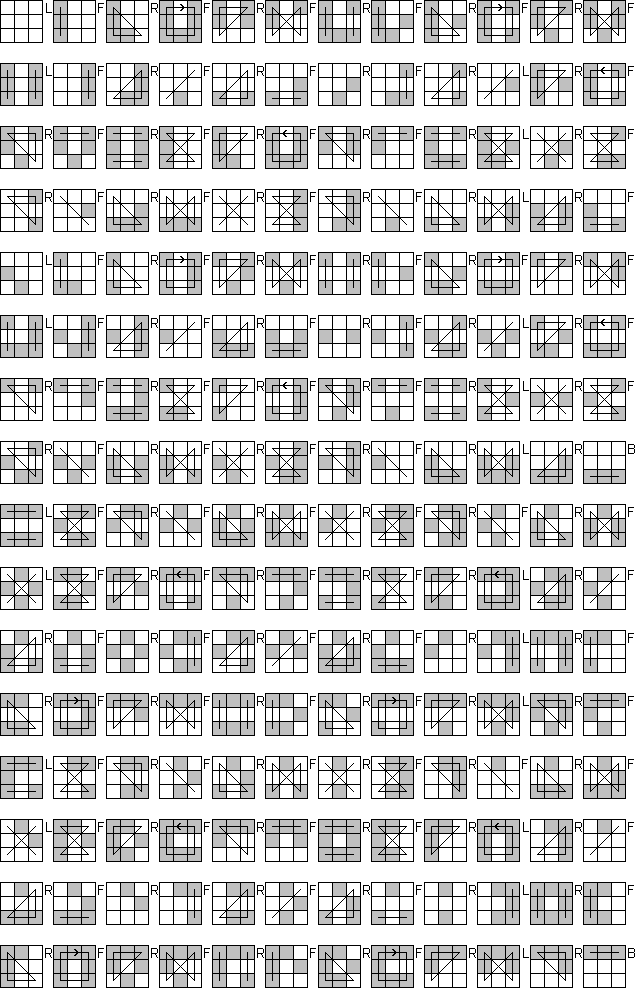# Hamilton Paths and Cycles on puzzles

I have always wondered whether it is possible to find a sequence of moves on the Rubik's Cube that visits every position exactly once. My attempts to answer this question form the basis of this page. Though this is of little or no practical value in the real world of puzzles, I hope you find it interesting. Some basic knowledge of group theory is assumed, but you can probably understand the gist of most things from the context.

## Koenigsberg's bridges and Eulerian paths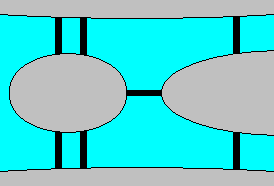Given the arrangement of 7 bridges between 4 landmasses shown on the right. Is it possible to devise a route that crosses each bridge exactly once? This was the arrangement of bridges in the city of Koenigsberg at the time Leonhard Euler solved the problem. He realised that if a landmass has an odd number of bridges leading from it, then the journey must start or end there. Every time you enter or leave a landmass, you use up one of its bridges. With an odd number of bridges, you must enter that landmass more often than leaving it, or vice versa. That can only mean you start there and end somewhere else, or end there but start somewhere else. In the case of Koenigsberg all four landmasses have an odd number of bridges, so no single route can cross every bridge.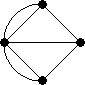The diagram of the city can be simplified to a graph of four vertices (the landmasses) and 7 edges (bridges) connecting them. An Eulerian path on a graph is a path that includes every edge exactly once. Euler proved that a graph must have no more than two vertices with an odd number of edges for there to be an Eulerian path. An Eulerian cycle or Eulerian circuit is an path that uses up every edge exactly once and also ends at the same vertex as it started. For an Eulerian cycle to exist, all the vertices of the graph must have an even number of edges.

The converse of these facts is also true, provided the graph is connected (i.e. does not consist of several separate subgraphs). If there are two odd vertices in a connected graph, then there is a path that goes along all the edges that starts and ends at those two vertices. If there are no odd vertices, then there is a path that goes along all the edges, and that path will have to start and end at the same vertex. These Eulerian paths or cycles are easy to find, because you can take any route you like provided that you make sure that you never do a move that would cause the untravelled edges to fall apart into two or more disconnected parts.

## Hamilton paths and Cayley graphs

So, it is completely known when there is a path or a cycle that uses up all the edges exactly once. Much less is known about when there is a path or a cycle that uses up all the vertices exactly once. A path or cycle that visits every vertex exactly once is called a Hamilton path or cycle. This is named after Sir William Hamilton, an Irish mathematician, who was the first to extensively examine them. Clearly any graph that is disconnected, or has a vertex that, were it and its edges removed, would make the graph disconnected, will not have a Hamilton cycle. Other than these and similarly obvious cases, graphs without a Hamilton cycle are remarkably rare.

In a puzzle context we also have graphs, in particular Cayley graphs. Suppose a puzzle's Cayley graph has a Hamilton path. This would correspond to a move sequence, sometimes called the Devil's Algorithm, that visits every possible position of the puzzle exactly once. Every move in the sequence creates a new position that hasn't been seen earlier on. Theoretically you could solve any position just by doing the move sequence until somewhere along the way it reaches the solved state. In reality this method is impractical of course because it would take too long.

Cayley graphs taken from permutation puzzles exhibit an extraordinary amount of symmetry. All the graph's vertices are the similar, because just by relabelling the pieces of the puzzle any position can be made to look like any other. In fact, this is not because of some property of the puzzle, but it is true for every Cayley graph of any group because all groups are permutation groups. More precisely, every Cayley graph is vertex-transitive - for any two vertices A and B, there is an isomorphism of the graph that maps A to B.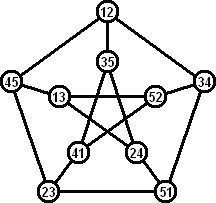The Lovász conjecture states that every vertex-transitive graph has a Hamilton path. Many mathematicians suspect this is false, though no counterexample is known. It is the case that there are vertex-transitive graphs that don't have a Hamilton cycle. The best known one is the Petersen graph, shown on the right. There are ten ways to choose two of the digits 1 to 5, and the ten vertices are labelled with such pairs in such a way that adjacent vertices have no digit in common. It is easy to see that this graph is vertex-transitive, just by relabelling the five digits. Less obvious is the fact that there is no Hamilton cycle. Suppose we have one edge of a path, and want to try to extend it to a Hamilton cycle. By relabelling, we can assume this first edge goes from 12 to 34. The next edge must go to 51 or 52, and by swapping labels 1 and 2 we can assume it goes to 51. The next edge after that must go to 24 or 23, and by swapping labels 3 and 4 we can assume it goes to 23. The vertices 24 and 52 each only have two available edges left, so any Hamilton cycle has to go through 41-52-13 and 13-24-35. This leaves vertex 45 with the edges 23-45-12, short-circuiting our path before it has visited all the vertices. This shows that a Hamilton cycle is impossible in the Petersen graph. It does have a Hamilton path.

Although the Petersen graph is vertex-transitive, it does not occur as a Cayley graph of some group. It has been conjectured that Cayley graphs always have a Hamilton cycle. If this is true, then every puzzle with a group structure has a Hamilton cycle. In particular, the puzzles with unrestricted reversible moves and distinct pieces, such as the 2×2×2 and 3×3×3 Rubik's Cubes, the Pyraminx, and the 12 colour Megaminx, will have Hamilton cycles.

From now on I will shorten the terms Hamilton path or cycle to Ham-path and Ham-cycle respectively.

## Lights Out and Gray codes

In the case of the Lights Out puzzle a Ham-path is actually easy to find. The classic Lights Out puzzle has 25 buttons/lights, but only 23 are needed to solve any position. Conversely, only 23 are needed to generate any position. If we think of those 23 buttons as on/off switches, every combination of the 23 on/off states of those switches will lead to a different position. A Ham-path will therefore go through all those on/off combinations by changing one switch at a time.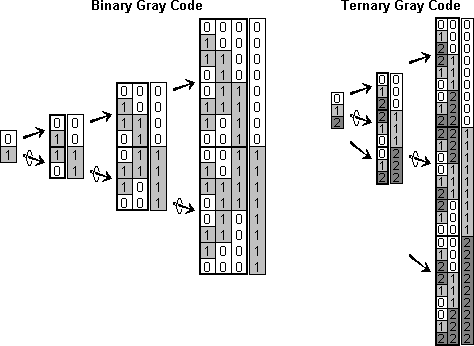If we had a path going through every combination of only 22 switches, we can easily extend it to all 23 switches as follows:
2. Go through every combination of the 22 on/off switches.
3. Turn the 23rd switch on.
4. Go backwards through every combination of the 22 on/off switches.

The path through every combination of 22 switches can be constructed in the same way from a path using only 21 switches, and so on recursively. This results in the standard binary Gray code, which was also seen in the Spinout, Brain, and Chinese Rings puzzles. Note that the Ham-path above can be extended to a Ham-cycle by just switching off the 23rd light at the end.

The binary Gray code is a Ham-path for Lights Out when every light has two states. Almost the same construction can be used to construct a ternary Gray code, and this gives a Ham-path for a 3-state Lights Out puzzle. To construct a Ham-path for n lights from a Ham-path for n-1 lights, do these steps:
2. Go through every state-pattern of the first n-1 lights.
3. Change light n to state 1.
4. Go backwards through every state-pattern of the first n-1 lights.
5. Change light n to state 2.
6. Go forwards again through every state-pattern of the first n-1 lights.
It is easy for 1 light (or even zero lights), a Ham-path code can be recursively constructed for any number of 3-state lights. Note that this cannot be extended to a Ham-cycle, but different methods of construction will lead to Ham-cycles, as we shall see later.

The Rubik's Clock is another puzzle where the order of the moves does not matter, so that the underlying group is Abelian. This time it is not binary or ternary, but base 12. The normal solving method uses (at most) 14 types of move. Any mixed position can therefore be denoted by how far the wheels have to turn in those 14 moves in order to solve it.

Suppose we construct a base 12 Gray code, of 14 digits. In other words, we construct a list of numbers in base 12, all with 14 digits, in such an order that two consecutive numbers in the list differ in only one digit. This will then be a Ham-path of the Rubik's Clock, where each set of the 14 digits signifies the current position as explained in the previous paragraph. Two consecutive numbers differ only at one digit, so those two positions are indeed connected by a single move.

These Gray codes work only in the case of an Abelian group, i.e. cases where the order in which you do the moves does not matter. Later on I will generalise this method a little bit to some non-Abelian cases.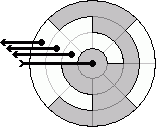Before moving on, I'll just digress a bit to describe one practical application of a binary Gray code. Many digital cameras have a small wheel for selecting the current mode (normal, video, portrait, landscape, etc.). Such a wheel usually has 8 modes to choose from. The position of the wheel can be signaled to the camera's processor by three wires, each carrying a voltage or not, so the wheel just acts as a switch on those three wires. Ideally only one wire changes its state as you go from one mode to the next, otherwise the processor might receive false signals when the changes on the several wires don't occur exactly simultaneously. This is exactly what a 3-bit binary Gray code does - it lists all eight possible states in such an order that two consecutive states differ by only one bit. The drawing on the right shows how such a selection wheel could be designed. My own digital camera's selector wheel has eight states too, but it recognises if the wheel is halfway between two options. This could be done with a 4-bit Gray code, where every signal with an odd number of bits set signifies an illegal in-between state.

## Bell-ringing

Suppose you have a simple puzzle consisting of n pieces in a row, and where the only moves you are allowed to do is to swap two adjacent pieces. This is of course an easy puzzle to solve, because once you have put piece n in its correct place it does not interfere with solving the remaining n-1 pieces. Is there a Hamilton cycle here, i.e. a sequence of n! moves such that each of the n! permutations is encountered when those moves are done?

It turns out there is such a sequence. If we have only 2 pieces, the cycle is almost trivial:

``````  1 2

2 1
``````

There are only two positions, and one swap brings you from one to the other, and then back again. For 3 pieces, start with the cycle for two pieces and replicate each line three times. Then weave through the third piece, to get this:

``````
1   2 3
1 3 2
3 1   2
3 2   1
2 3 1
2   1 3
``````

Clearly each shift of piece 3 is an adjacent swap, i.e. a legal move of our puzzle. Furthermore, this is still a cycle, as the last and first positions differ by a single move. This can be extended to 4 pieces - replicate each line four times, and weave piece 4 back and forth between them. This extends up to any number of pieces n.

This method was described in the 17th century, as it applies to bell-ringing in which a number of bells are rung, each time in a different sequence. This system of swapping adjacent bells, called plain changes, was feasible to do in practice, and produces all possible bell sequences.

To me this has a very similar feel to the way Gray code was generated in the earlier examples. I think it is only a superficial similarity, since little else carries over from that setting to this case.

For any puzzle where adjacent swaps are allowed, we have found a Hamilton path regardless of the number of pieces of the puzzle. There is a reason why this worked, and why this is not so easily adapted for other kinds of puzzle. When an extra piece was added, it could be woven through; i.e. it could visit all possible locations in turn while leaving the rest of the pieces in the same order after it had passed by. Those other pieces were shifted over to one side or other, but that did not matter since the same moves are possible on locations 1 to n-1 as on locations 2 to n. This is sadly not so in any genuine puzzles I know of.

## Topspin

The one puzzle in which it is tempting to try something of this sort is Topspin, where each turn of the turntable is a move, but shifts of the loop are not. Let's consider a section of the loop, n pieces long. If we rearrange the pieces in that section alone then only even permutations are possible. Odd permutations can only be realised by disturbing the rest of the pieces too. Is it possible to weave piece n+1 through each spot between pieces 1 to n? If n is a multiple of 4, then there is:

``````
1234x
1x432
34x12
321x4
x1234
``````

It doesn't matter that the other pieces are temporarily rearranged, as long as they are restored once the new piece has finished weaving through. This weaving pattern shows that if we have a Hamilton cycle for 4k pieces, then it can be extended to 4k+1 pieces. It is unfortunately not possible to the same for other numbers of pieces, so we cannot generate Hamilton paths this way in the general case. Clearly a different approach is needed here. While the idea of weaving a piece through the others is a nice one, it turns out that it is only very rarely useful.

## Paths and cycles in direct products of groups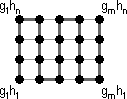The Gray code construction method works generally for direct products of Hamilton groups. Suppose G is a group with a Ham-path g1, g2, ..., gn, and H is a group with a Ham-path h1, h2, ..., hm. The direct product G×H then has the path (g1,h1), (g1,h2), ..., (g1,hm), (g2,hm), (g2,hm-1), ..., (g2,h1), (g3,h1),... etc. The path goes back and forth through the path in H, each time with the next element on the path in G. If you think of the indices as coordinates, this is simply a path zigzagging through all vertices in an m by n rectangular grid graph.

Obviously this method can be repeated, to get a path for the product of any finite number of Hamilton groups. Any finite Abelian group is the direct product of cyclic groups, and cyclic groups have an obvious Ham-path. Therefore, every Abelian group has a Ham-path too. This is exactly the Gray code method described in the previous section. Remember however that the path construction for G×H works even when G and H are not Abelian as long as they have Hamilton paths.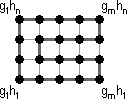There are other paths through every vertex in a rectangular grid. With a little care we can usually find a Ham-cycle, not just a Ham-path. If one of G or H has an even number of elements, then there is always a Ham-cycle as shown here.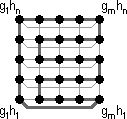This diagram shows a cycle in GxH if both G and H have odd order, and one of them (say G) has a Ham-cycle and the other only a Ham-path. Only if both G and H are odd and merely have Ham-paths but no cycles, then it is not possible to get a cycle in this way (easily proved by a checkerboard colouring). This does not prove that there are no Ham-cycles in that case; merely that this method is not able to produce one.

One example of a permutation puzzles which has a group that splits into direct products is the Pyraminx. Turning the small tips of the Pyraminx doesn't affect the rest of the puzzle. If we have a Ham-path for the Tetraminx, i.e. for the body of the Pyraminx, then from the above it follows that we can easily extend it to a Ham-cycle of the whole Pyraminx including the small tips.

Things are not always so simple. Implicit in all the above is that G and H are completely separate. In other words, following the path in G is possible without affecting the H coordinate, and vice versa. The generators we have for G have no effect on the elements of H. The thing is, we don't have a choice about these generators - they are the moves of the puzzle. Often the puzzle's group does split into a direct product, but all the generators are non trivial in both coordinates. The Megaminx for example has a group that is a direct product of the group of corner positions and the group of edge positions. Any attainable corner arrangement (ignoring edges) and any attainable edge arrangement (ignoring corners) combine to an attainable Megaminx arrangement. The only available moves affect both corners and edges, so Ham-paths of the two subgroups don't easily combine into a Ham-path of the whole group. Furthermore we cannot separate the available moves into two sets, one for the edges and one for the corners.

Suppose on the other hand that the generators for H don't affect elements of G, but the generators for G do affect H. In this case the zigzag method does still work. The reason if that if h1, h2, ..., hm is a Ham-path of group H, then for any h in H, the sequence hh1, hh2, ..., hhm is also a Ham-path. In other words, if a move sequence applied to the start position visits every possible position, then that move sequence applied to some mixed position will also visit every possible position. The zigzag method of constructing a ham-path on G×H still allows you to visit each H coordinate after each step in the G coordinate.

This can be applied to the Pyraminx/Tetraminx to simplify things further. If you turn the top vertex, i.e. do move U, three edges are of course moved with it. The top vertex piece may also be twisted in isolation, though it takes a few moves to do so. Turns of the other three vertices don't affect the top vertex piece however. We can therefore split off twists of the top vertex piece. Suppose P is a move sequence that involves only turns of the three non-U vertices, and that P visits every possible position involving the edges and those three vertex pieces. In other words, P is a Ham-path of the Pyraminx if the top vertex piece is not affected and also ignored. Then P U P U P is a Ham-path of the whole Pyraminx.

We still need to find P, but its length is about a third of the complete path we were looking for at first. It is also more constrained since it uses only three types of moves instead of four, but whether this would make it easier or harder to find is not clear to me. We cannot do the same trick again to split off another vertex piece, since it is not possible to use turns of the remaining two vertices to generate all the edge positions. You cannot flip any edges for example with just two types of move.

## Paths in coset spaces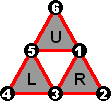The Pyraminx is a little too complicated an example, so let's examine a simplified version without the vertex pieces nor the edge orientations. It has three faces, each with three pieces, and any two faces share exactly one piece. This is illustrated on the right.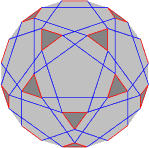Suppose we use turns of only two of the faces. The two faces have five pieces all together, and only even permutations are possible, giving 5!/2 = 60 possible positions. This puzzle is small enough for us to draw its Cayley graph. The graph will of course have 60 vertices, and each vertex has four edges corresponding to the moves L, L', R, and R'. One way to show this particular graph in a very symmetric way is on the surface of a truncated dodecahedron, shown on the right. Though visually quite nice, it does not give much insight into a Hamilton path, except the clue that it might be possible to make use of the five-fold symmetry. A different version of the same graph is shown below, again with five-fold symmetry.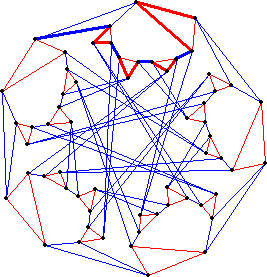A number of edges is drawn thicker than the rest. Repeating that section in each of the five identical parts of the graph results in a cycle that visits every vertex, i.e. it is a Hamilton cycle. This gives us the Ham-cycle (R'R'LRRL'RRLRRL)5. Below is a table that lists the order in which the cycle visits all the positions of this puzzle.

R' R' L R R L' R R L R R L 12345 23145 31245 31524 53124 15324 15243 21543 52143 52314 35214 23514 23451 34251 42351 42135 14235 21435 21354 32154 13254 13425 41325 34125 34512 45312 53412 53241 25341 32541 32415 43215 24315 24531 52431 45231 45123 51423 14523 14352 31452 43152 43521 54321 35421 35142 13542 51342 51234 12534 25134 25413 42513 54213 54132 15432 41532 41253 24153 12453

Suppose we traverse this cycle twice. Somewhere during the first cycle we make a note of one of the positions, and obviously 60 moves later during the second cycle we well see that same position again. Between those two visits every other position is visited once, so the moves done between those two visits is also a Ham-cycle. In other words any cyclic rearrangement of the Ham-cycle is also a Ham-cycle. For example (LRRL'RRLRRLR'R')5 is a cyclic rearrangement (the first two R' moves have been shifted to the other end), and is therefore also a Ham-cycle. This feature of Ham-cycles in Cayley graphs is very useful as you will see in a moment.

If we remove the last edge of a Ham-cycle, we get a Ham-path. We can reach the last vertex of this path from the first one either by following the whole path, or by traversing the removed edge in the opposite direction. In particular, if we leave the last move off the cycle (R'R'LRRL'RRLRRL)5, we get a move sequence that visits every position and has the same effect as L'. The last move of the cycle, however, need not be L, as we can use any cyclic rearrangement of the move sequence. Note that our cycle uses all four possible moves, L, L', R, and R', so we can make Ham-paths that have the same effect as any of those moves.

Let's now extend the small example to use the third face and include the sixth piece. Given a particular piece in the sixth position, we can use one of our Ham-paths to visit all such arrangements. Therefore the idea is to put each piece in turn into that sixth location, and apply a Ham-path in between, thus visiting every possible position. What remains for us to do is to find a sequence of moves that alternates a U or U' move by some other move, and which puts a new piece in the sixth location after each U or U'.

This is not difficult. For example, (RU)4LU.

 RU RU RU RU LU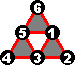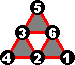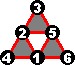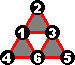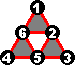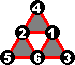Now we replace each R in this sequence by (LRRL'RRLRRLR'R')4LRRL'RRLRRLR', the Ham-path we found that has the same effect, and similarly replace the L by (RRLRRLR'R'LRRL')4RRLRRLR'R'LRR. We must also append a Ham-path in order to visit all positions with piece 4 at the top. The result is a move sequence that is 359 moves long and which is a Ham-path for the whole puzzle:

[(LRRL'RRLRRLR'R')4 LRRL'RRLRRLR' U ]4 (RRLRRLR'R'LRRL')4 RRLRRLR'R'LRR U (RRLRRLR'R'LRRL')4 RRLRRLR'R'LRR

Let's very briefly put this in a group theory setting. The puzzle group G has a subgroup H, in this case the subgroup generated by two of the three faces. H has a Ham-cycle that uses all of the generators of H at least once. The subgroup H has right cosets, c1H ... cnH, where in our case the coset representatives ci are the six positions shown above. Those are chosen such that ci+1 = ci·hi·gi, for some gi, one of the generators of G, and hi, one of the generators of H. It is the gi that form the edges between the cosets. Then we replaced each hi by an equivalent Ham-path of H, in order to visit every element of the coset ciH, and also appended some Ham-path of H for visiting the elements of last coset cnH.

## Making use of commutating moves

In the previous example it was easy to find the path through all the cosets, because there were only 6 of them. It can be quite difficult when there are many cosets to find a path between. Another problem is that sometimes there simply is no such path because of the restriction that each step from one coset to the next must use exactly two generators. For example, let's take another look at Topspin.

Suppose we have a sequence of moves for Topspin which cycles through all the (even) permutations of 8 pieces. We would like to extend that to include a ninth piece. Applying the method from the previous section we need to put each piece into the ninth position one by one, but we are only allowed two moves per piece. This is clearly impossible to achieve, since the piece at location 1 cannot possibly be moved all the way to location 9 in two twists of the turntable. The only way out is to get rid of this restriction. We can do this in two ways. Firstly, instead of using a Ham-cycle to generate Ham-paths that have the same effect as a single move, we could try to find Ham-paths for the first 8 pieces which have a different effect than a single move. The effects of such Ham-paths would allow us to bring any piece into the ninth position with one further move. This is rather difficult, and has the problem that the final result is a Ham-path rather than a cycle, so you cannot use the same technique to then add further pieces into the mix. The second way to do this is much more useful, and makes use of commuting moves to visit the positions in a less rigidly prescribed order. It is more easily explained by a few examples.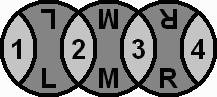Let's take the simple puzzle consisting of a row of 4 pieces, where a move is a swap of two adjacent pieces. Lets call those moves L, M, and R (Left, Middle, Right). There is a Ham-cycle of the permutations of pieces 1-3, namely LMLMLM, which visits the positions 123, 213, 231, 321, 312, 132, 123, (piece 4 not shown). By leaving off the last M move we have a Ham-path LMLML that has the same effect as M. Following the technique of the previous section, we intersperse R moves that put a new pieces at the rightmost position with the Ham-path LMLML that does all permutations of the other pieces and puts a new piece ready to be picked up by the R move that follows it. This results in: (LMLML R)3. Unfortunately it repeats after the third time because (LMLML R)3 = (MR)3 = I, and this never puts piece 1 in the rightmost position. Below is a table that shows this sequence and the positions it visits.

L M L M L R 1234 2134 2314 3214 3124 1324 1342 3142 3412 4312 4132 1432 1423 4123 4213 2413 2143 1243

We also have the Ham-path MLMLM that visits all permutations of 1-3 and has the same effect as the move L. The move sequence R MLMLM R has the same effect as RLR, and as L and R commute, this is the same as a single L move. Looking through the table above we find that there are a few places where an L move is done on a position with piece 1 in the third place. By replacing that L move by R MLMLM R we can then also visit all positions that have piece 1 in the rightmost position. This leads to the Ham-cycle (LMLML R)2 LM(R MLMLM R)ML R.

 L M L M L 1234 2134 2314 3214 3124 1324 1342 3142 3412 4312 4132 1432 1423 4123 4213 2413 2143 1243 4231 4321 3421 3241 2341 2431

## A Ham-cycle for the Floppy cube

Using the methods discussed so far we can find a Ham-cycle for the Floppy Cube. It has 4 edge pieces which don't move but can be flipped, and 4 corner pieces which permute. It is identical to the Rubik's Cube subgroup <F2, B2, R2, L2>. Quarter turns are not possible on the Floppy Cube so I will denote the group as <F, B, R, L>, or just G from now on. This puzzle has 192 positions.

Firstly, let's halve the size of the problem. The subgroup G2 = <F, R, L> is half the size of G, and contains the 96 positions in which the edge on the B side is not flipped. Suppose we have a Ham-path X for this subgroup. Then X B X is a Ham-path for the full group G: The first X visits all positions with the B edge unflipped, B flips that edge, and then the second X visits all positions with that B flipped. If we make sure that X is a Ham-path that has the same effect as F, then we can go even further and get the Ham-cycle X B X B.

To get this X, let's now try to find a Ham-cycle for the smaller group G2. We can try the same trick again, removing the L move and just looking at the even smaller group G3 = <F, R>. This group has 12 elements and has a nice Ham-cycle, namely (FR)6. Notice that this is very similar to the example in the previous section, with four pieces (corners) in a row and any two adjacent ones can be swapped. The only difference is that there are now edge pieces with an orientation, and that is why this G3 Ham-cycle is 12 moves long instead of 6. From this we get the two G3 Ham-paths RFRFRFRFRFR and FRFRFRFRFRF, which have the same effect as F and R respectively.

Within the group G2, G3 has 8 cosets. Each coset is a set of 12 positions that have the same corner piece at the back-left, and the same left-edge orientation. Alternating the F Ham-path with an L move we get the move sequence (RFRFRFRFRFR L)6 which traverses six of those cosets before repeating. It happens for the same reason as before: The L moves in the sequence (RFRFRFRFRFR L)6 = (FL)6 never move the back-right corner to the back-left location. We now merely have to look through the positions and find one where an R move is done while the back-right corner piece is at the front-left location, ready to be slotted into the back-left with an L move. At that point we can replace the R move by L FRFRFRFRFRF L to get the sequence to traverse one of the missing cosets.

Now let's put things together. In the move sequence (RFRFRFRFRFR L)6, the first two moves put the back-right corner at the front-left. The R move immediately following that is therefore the first that can be replaced. This gives the move sequence RF(L FRFRFRFRFRF L)FRFRFRFR L (RFRFRFRFRFR L)5 which covers 7 of the 8 cosets. To cover the other missing coset we want to find an R move in the sequence during which the back-right corner is again at the front-left, but now with the left edge flipped. As (RFRFRFRFRFR L)3 = (FL)3 flips the left and front edges and does nothing else, the positions visited in the second half of (RFRFRFRFRFR L)6 are the same as the first half, except for those edge flips. To cover the second missing coset we replace the same R move as in the first half, giving the sequence Y = (RF(L FRFRFRFRFRF L)FRFRFRFR L (RFRFRFRFRFR L)2)2.

The sequence Y is a Ham-cycle for G2 = <F, R, L>. We'd like it to start with an F move, and we can do this by shifting the first R move to the end of the sequence, giving FPFP, where P = (L FRFRFRFRFRF L)FRFRFRFR L (RFRFRFRFRFR L)2) R. Therefore PFP is a Ham-path for G2 that has the same effect as an F move. At last we can then construct the Ham-cycle for to whole group by alternating it with a B move, which results in PFPB PFPB.